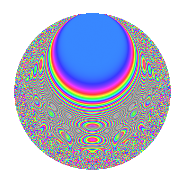# Properties

 Label 2009.2.aLevel 2009 Weight 2 Character orbit a Rep. character $$\chi_{2009}(1,\cdot)$$ Character field $$\Q$$ Dimension 136 Newforms 21 Sturm bound 392 Trace bound 3

# Related objects

## Defining parameters

 Level: $$N$$ = $$2009 = 7^{2} \cdot 41$$ Weight: $$k$$ = $$2$$ Character orbit: $$[\chi]$$ = 2009.a (trivial) Character field: $$\Q$$ Newforms: $$21$$ Sturm bound: $$392$$ Trace bound: $$3$$ Distinguishing $$T_p$$: $$2$$, $$3$$

## Dimensions

The following table gives the dimensions of various subspaces of $$M_{2}(\Gamma_0(2009))$$.

Total New Old
Modular forms 204 136 68
Cusp forms 189 136 53
Eisenstein series 15 0 15

The following table gives the dimensions of the cuspidal new subspaces with specified eigenvalues for the Atkin-Lehner operators and the Fricke involution.

$$7$$$$41$$FrickeDim.
$$+$$$$+$$$$+$$$$29$$
$$+$$$$-$$$$-$$$$37$$
$$-$$$$+$$$$-$$$$41$$
$$-$$$$-$$$$+$$$$29$$
Plus space$$+$$$$58$$
Minus space$$-$$$$78$$

## Trace form

 $$136q - 2q^{2} + 136q^{4} + 2q^{6} - 6q^{8} + 132q^{9} + O(q^{10})$$ $$136q - 2q^{2} + 136q^{4} + 2q^{6} - 6q^{8} + 132q^{9} - 6q^{11} - 8q^{12} - 12q^{13} - 2q^{15} + 120q^{16} - 8q^{17} - 22q^{18} - 4q^{19} + 16q^{20} - 8q^{22} - 12q^{23} + 26q^{24} + 124q^{25} + 8q^{26} - 6q^{27} - 8q^{29} + 10q^{30} + 8q^{31} - 14q^{32} - 4q^{33} + 140q^{36} + 16q^{37} + 26q^{38} - 16q^{39} + 20q^{40} - 4q^{41} - 8q^{43} - 38q^{44} + 32q^{45} + 20q^{46} + 4q^{48} - 6q^{50} - 32q^{51} - 36q^{52} + 4q^{53} + 30q^{54} - 18q^{55} - 4q^{57} - 32q^{58} - 16q^{59} + 34q^{60} - 4q^{61} + 80q^{64} + 4q^{65} + 96q^{66} + 6q^{67} + 32q^{68} + 32q^{69} - 12q^{71} - 50q^{72} - 52q^{73} - 16q^{74} + 24q^{75} + 24q^{76} - 24q^{78} - 24q^{79} + 36q^{80} + 96q^{81} - 2q^{82} + 4q^{83} - 44q^{85} + 36q^{86} - 4q^{87} + 28q^{88} + 44q^{89} - 32q^{90} + 20q^{92} + 8q^{93} + 42q^{94} - 18q^{95} + 46q^{96} - 36q^{97} - 24q^{99} + O(q^{100})$$

## Decomposition of $$S_{2}^{\mathrm{new}}(\Gamma_0(2009))$$ into irreducible Hecke orbits

Label Dim. $$A$$ Field CM Traces A-L signs $q$-expansion
$$a_2$$ $$a_3$$ $$a_5$$ $$a_7$$ 7 41
2009.2.a.a $$2$$ $$16.042$$ $$\Q(\sqrt{5})$$ None $$-1$$ $$1$$ $$-1$$ $$0$$ $$-$$ $$-$$ $$q-\beta q^{2}+(1-\beta )q^{3}+(-1+\beta )q^{4}+(-1+\cdots)q^{5}+\cdots$$
2009.2.a.b $$2$$ $$16.042$$ $$\Q(\sqrt{5})$$ None $$-1$$ $$3$$ $$1$$ $$0$$ $$-$$ $$+$$ $$q-\beta q^{2}+(1+\beta )q^{3}+(-1+\beta )q^{4}+(1+\cdots)q^{5}+\cdots$$
2009.2.a.c $$2$$ $$16.042$$ $$\Q(\sqrt{13})$$ None $$0$$ $$-1$$ $$1$$ $$0$$ $$-$$ $$-$$ $$q-\beta q^{3}-2q^{4}+(1-\beta )q^{5}+\beta q^{9}+(-2+\cdots)q^{11}+\cdots$$
2009.2.a.d $$2$$ $$16.042$$ $$\Q(\sqrt{13})$$ None $$0$$ $$1$$ $$-1$$ $$0$$ $$+$$ $$+$$ $$q+\beta q^{3}-2q^{4}+(-1+\beta )q^{5}+\beta q^{9}+\cdots$$
2009.2.a.e $$2$$ $$16.042$$ $$\Q(\sqrt{5})$$ None $$1$$ $$-2$$ $$2$$ $$0$$ $$-$$ $$-$$ $$q+\beta q^{2}-q^{3}+(-1+\beta )q^{4}+q^{5}-\beta q^{6}+\cdots$$
2009.2.a.f $$2$$ $$16.042$$ $$\Q(\sqrt{5})$$ None $$1$$ $$2$$ $$-2$$ $$0$$ $$+$$ $$+$$ $$q+\beta q^{2}+q^{3}+(-1+\beta )q^{4}-q^{5}+\beta q^{6}+\cdots$$
2009.2.a.g $$3$$ $$16.042$$ 3.3.148.1 None $$-1$$ $$0$$ $$2$$ $$0$$ $$-$$ $$+$$ $$q+(-\beta _{1}-\beta _{2})q^{2}-\beta _{2}q^{3}+(1+2\beta _{1}+\cdots)q^{4}+\cdots$$
2009.2.a.h $$3$$ $$16.042$$ 3.3.257.1 None $$0$$ $$-3$$ $$0$$ $$0$$ $$-$$ $$+$$ $$q-\beta _{2}q^{2}+(-1-\beta _{2})q^{3}+(1+\beta _{1}-\beta _{2})q^{4}+\cdots$$
2009.2.a.i $$3$$ $$16.042$$ 3.3.257.1 None $$0$$ $$3$$ $$0$$ $$0$$ $$-$$ $$-$$ $$q-\beta _{2}q^{2}+(1+\beta _{2})q^{3}+(1+\beta _{1}-\beta _{2})q^{4}+\cdots$$
2009.2.a.j $$3$$ $$16.042$$ 3.3.257.1 None $$1$$ $$1$$ $$-6$$ $$0$$ $$-$$ $$-$$ $$q+\beta _{1}q^{2}+(\beta _{1}-\beta _{2})q^{3}+(1+\beta _{2})q^{4}+\cdots$$
2009.2.a.k $$3$$ $$16.042$$ $$\Q(\zeta_{14})^+$$ None $$4$$ $$-5$$ $$-2$$ $$0$$ $$-$$ $$+$$ $$q+(1+\beta _{1})q^{2}+(-2+\beta _{1})q^{3}+(1+2\beta _{1}+\cdots)q^{4}+\cdots$$
2009.2.a.l $$5$$ $$16.042$$ 5.5.233489.1 None $$-2$$ $$-2$$ $$2$$ $$0$$ $$+$$ $$+$$ $$q-\beta _{2}q^{2}-\beta _{3}q^{3}+(1+\beta _{1})q^{4}+\beta _{3}q^{5}+\cdots$$
2009.2.a.m $$5$$ $$16.042$$ 5.5.233489.1 None $$-2$$ $$2$$ $$-2$$ $$0$$ $$-$$ $$-$$ $$q-\beta _{2}q^{2}+\beta _{3}q^{3}+(1+\beta _{1})q^{4}-\beta _{3}q^{5}+\cdots$$
2009.2.a.n $$5$$ $$16.042$$ 5.5.633117.1 None $$-1$$ $$-4$$ $$5$$ $$0$$ $$-$$ $$-$$ $$q-\beta _{1}q^{2}+(-1+\beta _{1})q^{3}+(1+\beta _{2})q^{4}+\cdots$$
2009.2.a.o $$6$$ $$16.042$$ 6.6.185257757.1 None $$-1$$ $$4$$ $$1$$ $$0$$ $$-$$ $$+$$ $$q-\beta _{1}q^{2}+(1-\beta _{2})q^{3}+(1+\beta _{2}+\beta _{3}+\cdots)q^{4}+\cdots$$
2009.2.a.p $$7$$ $$16.042$$ $$\mathbb{Q}[x]/(x^{7} - \cdots)$$ None $$-1$$ $$-7$$ $$-4$$ $$0$$ $$-$$ $$-$$ $$q+\beta _{2}q^{2}+(-1+\beta _{1})q^{3}+(1-\beta _{2}+\beta _{4}+\cdots)q^{4}+\cdots$$
2009.2.a.q $$7$$ $$16.042$$ $$\mathbb{Q}[x]/(x^{7} - \cdots)$$ None $$-1$$ $$7$$ $$4$$ $$0$$ $$-$$ $$+$$ $$q+\beta _{2}q^{2}+(1-\beta _{1})q^{3}+(1-\beta _{2}+\beta _{4}+\cdots)q^{4}+\cdots$$
2009.2.a.r $$17$$ $$16.042$$ $$\mathbb{Q}[x]/(x^{17} - \cdots)$$ None $$3$$ $$-1$$ $$1$$ $$0$$ $$-$$ $$+$$ $$q+\beta _{1}q^{2}+\beta _{12}q^{3}+(1+\beta _{2})q^{4}+\beta _{13}q^{5}+\cdots$$
2009.2.a.s $$17$$ $$16.042$$ $$\mathbb{Q}[x]/(x^{17} - \cdots)$$ None $$3$$ $$1$$ $$-1$$ $$0$$ $$+$$ $$-$$ $$q+\beta _{1}q^{2}-\beta _{12}q^{3}+(1+\beta _{2})q^{4}-\beta _{13}q^{5}+\cdots$$
2009.2.a.t $$20$$ $$16.042$$ $$\mathbb{Q}[x]/(x^{20} - \cdots)$$ None $$-2$$ $$-8$$ $$-8$$ $$0$$ $$+$$ $$+$$ $$q-\beta _{1}q^{2}-\beta _{9}q^{3}+(1+\beta _{2})q^{4}-\beta _{18}q^{5}+\cdots$$
2009.2.a.u $$20$$ $$16.042$$ $$\mathbb{Q}[x]/(x^{20} - \cdots)$$ None $$-2$$ $$8$$ $$8$$ $$0$$ $$+$$ $$-$$ $$q-\beta _{1}q^{2}+\beta _{9}q^{3}+(1+\beta _{2})q^{4}+\beta _{18}q^{5}+\cdots$$

## Decomposition of $$S_{2}^{\mathrm{old}}(\Gamma_0(2009))$$ into lower level spaces

$$S_{2}^{\mathrm{old}}(\Gamma_0(2009)) \cong$$ $$S_{2}^{\mathrm{new}}(\Gamma_0(41))$$$$^{\oplus 3}$$$$\oplus$$$$S_{2}^{\mathrm{new}}(\Gamma_0(49))$$$$^{\oplus 2}$$$$\oplus$$$$S_{2}^{\mathrm{new}}(\Gamma_0(287))$$$$^{\oplus 2}$$